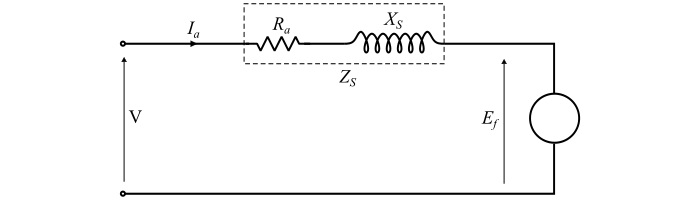# Equivalent Circuit of a Synchronous Motor

A synchronous motor is a doubly-excited system, i.e., it is connected to two electrical systems −

• A 3-phase supply is connected to the armature winding.

• A DC supply is connected to the rotor field winding.

The figure shows the equivalent circuit for one phase of a three-phase synchronous motorLet

• V = Terminal voltage per phase applied to the motor

• Ef = Excitation voltage

• Ia = Armature current per phase drawn by the motor from the supply

• Ra = Effective armature resistance per phase

• XS = Synchronous reactance per phase of the motor armature winding

• ZS = Synchronous impedance per phase of the armature

By applying KVL in the equivalent circuit of the motor, we have,

$$\mathrm{V=E_{f}+I_{a}Z_{s}\:\:\:\:\:\:...(1)}$$

The synchronous impedance of the motor is

$$\mathrm{Z_{S}=R_{a}+jX_{S}\:\:\:\:\:\:...(2)}$$

From eqns. (1) & (2), we get,

$$\mathrm{V=E_{f}+I_{a}(R_{a}+jX_{S})\:\:\:\:\:\:...(3)}$$

$$\mathrm{\Longrightarrow\:E_{f}=V-I_{a}R_{a}-jI_{a}X_{S}\:\:\:\:\:\:...(4)}$$

Where,

• IaRais the voltage drop per phase in the armature resistance.

• IaXSis the reactive voltage drop per phase due to armature reactance and armature reaction effect.

Also, the armature current per phase is given by,

$$\mathrm{I_{a}=\frac{V-E_{f}}{Z_{S}}\:=\frac{E_{r}}{Z_{S}}\:=\frac{E_{r}}{R_{a}+jX_{S}}\:\:\:\:\:\:...(5)}$$

Here, Eris the resultant voltage in the armature circuit.

The equivalent circuit of the synchronous motor helps considerably in understanding the operation of the motor as follows −

• If the field excitation is such that E= V, then the synchronous motor is said to be normally excited.

• If the field excitation is such that E< V, then the synchronous motor is said to be under-excited.

• If the field excitation is such that E> V, then the synchronous motor is said to be over-excited.